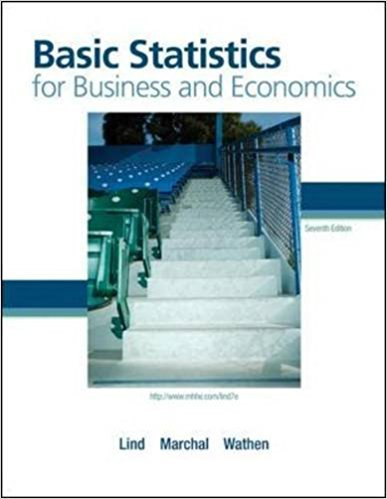×
×

# An investor purchased 100 shares of Fifth Third Bank stockISBN: 9780077384470 64

## Solution for problem 64E Chapter 5

Basic Statistics for Business and Economics | 7th Edition

• Textbook Solutions
• 2901 Step-by-step solutions solved by professors and subject experts
• Get 24/7 help from StudySoup virtual teaching assistantsBasic Statistics for Business and Economics | 7th Edition

4 5 0 421 Reviews
19
5
Problem 64E

An investor purchased 100 shares of Fifth Third Bank stock and 100 shares of Santee Electric Cooperative stock. The probability the bank stock will appreciate over a year is .70. The probability the electric utility will increase over the same period is .60.

a. What is the probability both stocks appreciate during the period?

b. What is the probability the bank stock appreciates but the utility does not?

c. What is the probability at least one of the stocks appreciates?

Step-by-Step Solution:
Step 1 of 3

Solution 64E

Step1 of 4:

The number of shares purchased an investor from Fifth Third Bank stock = 100,

The number of shares purchased an investor from Electric Cooperative stock = 100,

The probability the bank stock will appreciate over a year = 0.70, and

The probability the electric utility will increase over the same period = 0.60.

Here our goal is:

a). We need to find the probability both stocks appreciate during the period.

b). We need to find the probability the bank stock appreciates but the utility does not.

c). We need to find the probability at least one of the stocks appreciates.

Step2 of 4:

a).

Let us define an event are:

A = The bank stock will appreciate over a year.

B = The electric utility will increase over the same period.

Now, The probability both stocks appreciate during the period is:Therefore, The probability both stocks appreciate during the period is 0.42.

Step3 of 4:

b).

The probability the bank stock appreciates but the utility does not is:...

Step 2 of 3

Step 3 of 3

##### ISBN: 9780077384470

Unlock Textbook Solution